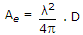# Electronics and Communication Engineering - Electromagnetic Field Theory

36.

A wavelength of 1 mm could be expected in

 A. VLF B. HF C. EHF D. SHF

Explanation:

No answer description available for this question. Let us discuss.

37.

The numerical value of directivity of current element of half-wave dipole is 1.76 and 2.15 dB respectively. The directive gain of half-wave dipole over current element will be

 A. 3.91 dB B. 1.95 dB C. 0.39 dB D. 0.195 dB

Explanation:

2.15 - 1.76 = 0.39 db.

38.

The maximum effective aperture of an antenna which is operating at wavelength of 2 m and has directivity of 100 is

 A. 15.92 B. 31.84 C. 2.18 D. 3.184

Explanation:39.

The waveguides compared to equivalent transmission lines at low frequency are

 A. able to carry more power B. less noisy C. having lower attenuation D. all of the above

Explanation:

No answer description available for this question. Let us discuss.

40.

The current distribution of a half wave dipole is

 A. uniform B. sinusoidal C. triangular D. complex